# Gateway Tests

Gateway tests are tests designed to determine the appropriate placement for students entering university, specifically if they are ready to embark on calculus university courses. They are intended to help the student, as opposed to something that would affect him or her negatively.

Below is a sample of such a test. If you score less than 8 out of 10, you should seriously re-consider doing calculus at university.

1. Solve the inequality |3x -7| < 4.

2. Simplify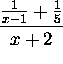.

3. Find all values of x for which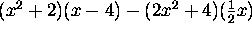is zero.

4. Find the points (a,b) on the graph of 2x+3y =6 that are (i) intercepts, (ii) have x = -3, (iii) have y=-2.

5. Find the equation of the line with slope 5 that passes through the point on the graph of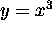with x-coordinate 2.

6. Find the equation of the line through the point (-1,3) that is parallel to the line 4x - 7y +6 = 0.

7. Find the equation of the line through the point (-1,3) that is perpendicular to the line 4x - 7y + 6 = 0.

8. If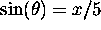and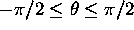, express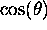in terms of x.

9. Sketch the graph of 2x -3y = 6.

10. Sketch the graph of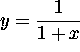.

We are always looking to improve our site. Please write if you have comments or suggestions.

Maintained by Edgar Goodaire, Department of Mathematics and Statistics, Memorial University of Newfoundland who last remembered to update the update date on July 20, 1999.

Design and graphics by Lori L. Barrett.